# Figure Matrix

In a Competitive exam, Reasoning is one of the most important section. A good score in Reasoning test can lead you to score very good marks in competitive exams. You can achieve a good marks only if you have a depth knowledge of reasoning skills. Basically reasoning is divided into few sections according to logic and type. This Figure Matrix topic is one of them.

"Representation of figures/elements following a certain rule or pattern, either rowwise or columnwise in a matrix from is know as Figure Matrix"

Questions based on 'Figure Matrix' generally have either a 2 x 2 or a 3 x 3 matrix. Each cell of this matrix contains a figure and there is a common pattern running through each of the rows or columns.

A candidate is required to analyse each of the sets to find out the common pattern and then identify the missing figure from the set of given alternatives, so as to complete the given matrix. The common pattern can be on the basis of number of elements, orientation of figure, addition or removal of elements etc.

## For clear understanding of this figure matrix, few examples are given below

Directions (Example Nos. 1-6)In each of the following questions, find out the answer figure which completes the problem figure matrix.

Example 1 In each of the following questions, find out which of the answer figures (a), (b), (c) and (d) completes the figure matrix ?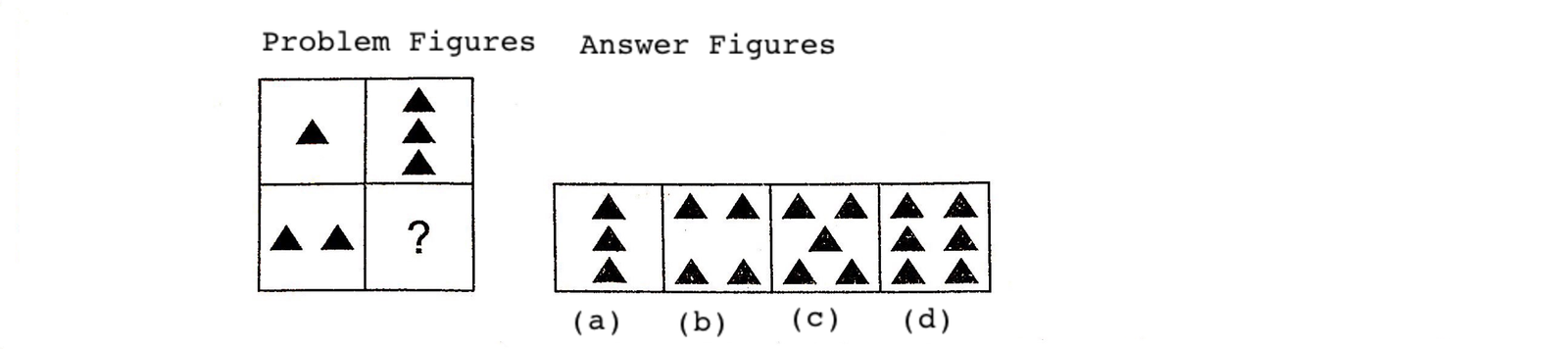Solution: (d) The second part of each row is thrice the first part. So, the figure given in option (d) will replace the question mark.

Example 2 In each of the following questions, find out which of the answer figures (a), (b), (c) and (d) completes the figure matrix ?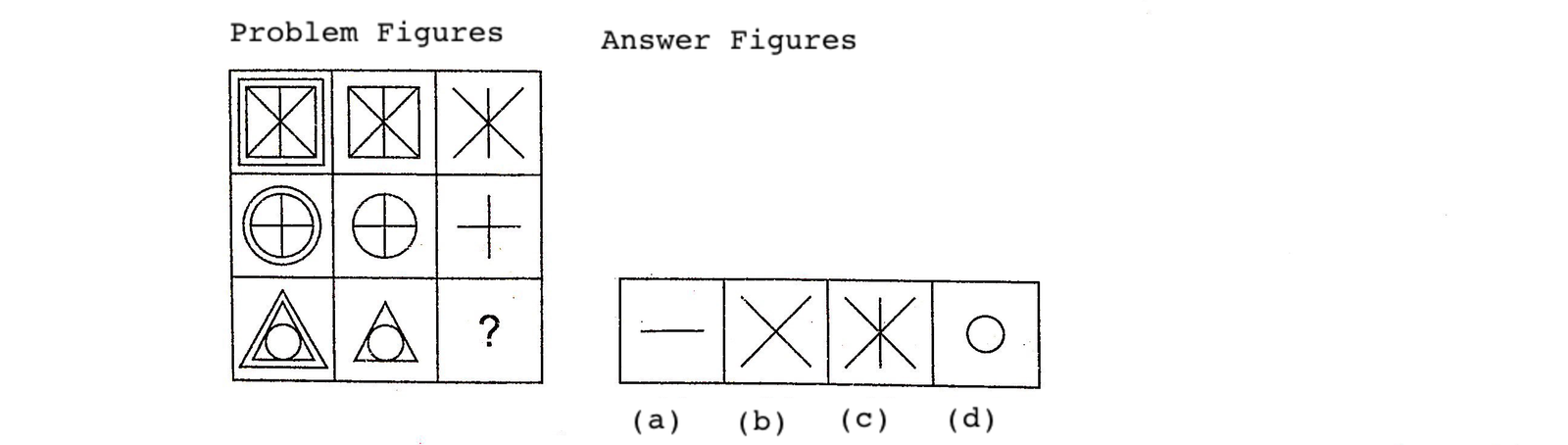Solution: (d) In each row, the second figure is obtained by removing the outer most element of the first figure and the third figure is obtained by removing the outer most element of the second figure.

Example 3 In each of the following questions, find out which of the answer figures (a), (b), (c) and (d) completes the figure matrix ?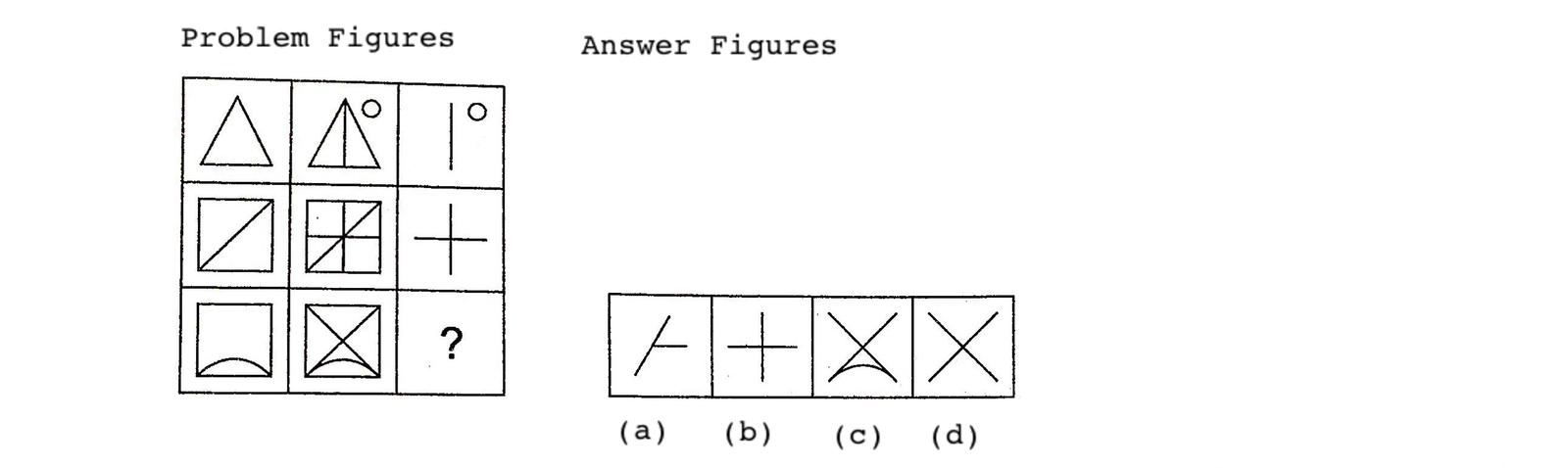Solution: (d) The third figure in each row comprises of parts which are not common to the first two figures.

Example 4 In each of the following questions, find out which of the answer figures (a), (b), (c) and (d) completes the figure matrix ?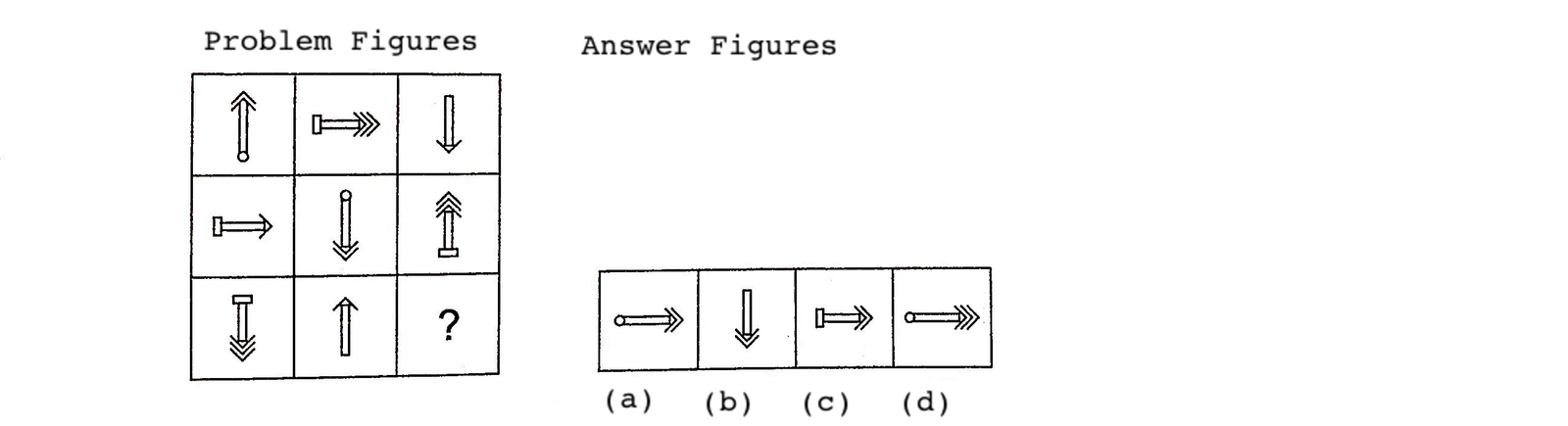Solution: (a) There are types of arrows --- a single-headed arrow, a double-headed arrow and a triple-headed arrow. There are three positions of arrows--Upwards, downwards and towards right. The arrows have 3 types of base-plane, rectangular and circular. Each of these features is used once in each row.

Example 5 In each of the following questions, find out which of the answer figures (a), (b), (c), (d) and (e) completes the figure matrix ?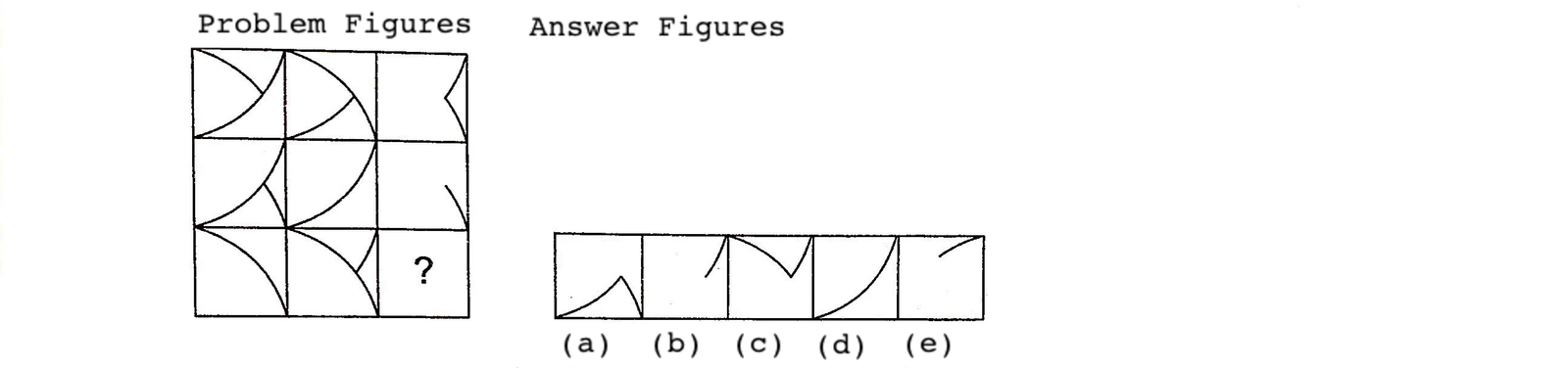Solution: (b)The contents of the third figure in each row (and column) are determined by the contents of the first two figures. Lines are carried forward from the first two figures to the third one, except where two lines appear in the same position, in which they are cancelled out.

Example 6 In each of the following questions, find out which of the answer figures (a), (b), (c),(d) and (e) completes the figure matrix ?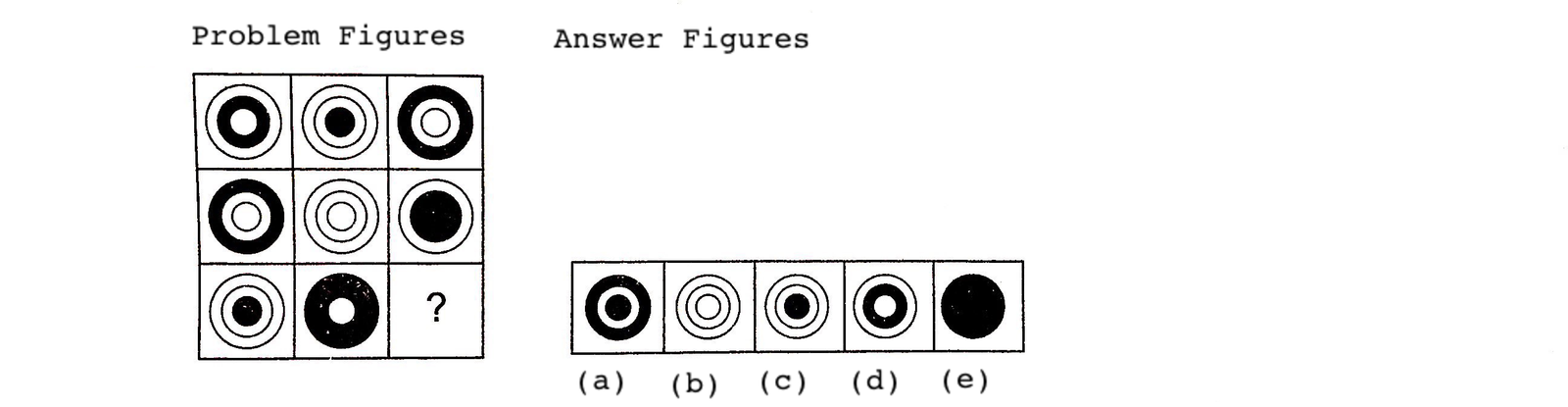Solution: (b)In each row (and column), the superimposition of all the three figures results in a darkened circle.

## TIPS on cracking Non Verbal Reasoning Questions on Figure Matrix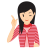Few Important things to Remember

Time is a very important thing in every exams. You should complete your exam within the time frame. But in a competitive exam, question are for testing your calculation ability within a given time frame. They tests, how fast you can solve a paper. That’s why so many students won’t complete their exam within time. But if you use tricks of reasoning and Figure Matrix then it will help you to solve bank, government or any other exam paper much faster.

Tip# : In this type of questions, more than one set of figures is given in the form of a matrix, all of them following the same rule. The candidate is required to analyse the complete sets; find out the common rule and then on its basis, find the missing figure in the incomplete set.

Question: Select a suitable figure from the four alternatives that would complete the figure matrix.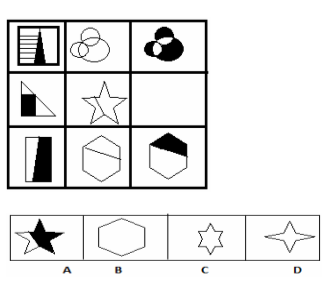Solution: (A)